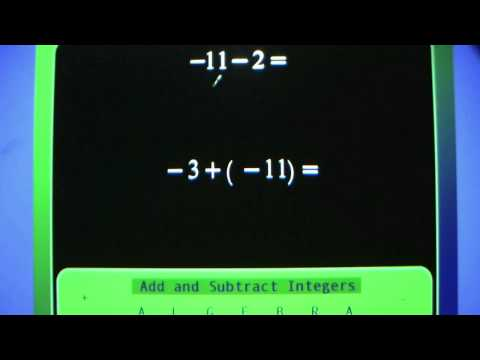## Integers

Subject: Compulsory Maths

#### Overview

The absolute value of an integer is the numerical value without power to whether the sign is negative or positive. An integer is a whole number (not a fractional number) that can be positive, negative, or zero.

#### Review on Integers

An integer is a whole number (not a fractional number) that can be positive, negative, or zero.
The set of integers is denoted by the letter 'Z'.
Z = {. . . . . . . . . . -3, -2, -1, 0, 1 , 2, 3 . . . . . . . . .} is the set of integers. i.e. both +ve and -ve.
Z+ = {+1, +2, +3, +4, +5, +6 . . . . . . . . .} is the set of +ve integers.
Z-= {-1, -2, -3, -4, -5, -6 . . . . . . . . . } is the set of -ve integers.

In the above number line, the negative integers left to the zero are increasing.
i.e. -3< -2< -1< 0< 1< 2< 3< 4 and so on.

#### Absolute Value of Integers

The absolute value of an integer is the numerical value without power to whether the sign is negative or positive. For example:
let A and B be two places in which A is -5 km left from zero and B is +5 km right from zero. Then, what can be the distance between A and B.
In simple sense,
Distance of A + Distance of B,
= -3 + 3
= 0, which is impossible.
But by using absolute value, we can write as:
Distance of (A + B) = |-3| + |3|
= 3 + 3
= 6 km.
In such case, the numerical value of either 3 or -3 will be same i.e. 3.

#### Operation on Integers

The fundamental operations of integers on number lines are:addition

• subtraction
• multiplication
• division

##### Things to remember
• An integer is a whole number (not a fractional number) that can be positive, negative, or zero.
• The absolute value of an integer is the numerical value without power to whether the sign is negative or positive.
• The set of integers is denoted by the letter 'Z'.
• It includes every relationship which established among the people.
• There can be more than one community in a society. Community smaller than society.
• It is a network of social relationships which cannot see or touched.
• common interests and common objectives are not necessary for society.
##### Videos for Integers##### Add and Subtract Integers (Important Algebra Skill)

Solution:

In addition, Here, (+4) + (+5)

= +(4+5) = +9 ans.

Again, In subtraction, Here, (+9) - (+4)

= + (5-4) = +5 ans.

Answer: The set of all the numbers both positive and negative including zero is called the set of integers.

Solution:

Here, (+5) + (-2)

= +(5-2) = +3

Solution:

Here,  (-4) + (-6)

= - (4+6) = -10

Solution:

Here, (-3) - (+8)

= -(3+8) = -11

Solution:

In Addition, Here, (+3) + (-7)

= (-7) + (+3)

= -(7-3) = -4 ans.

Again, Here, (+5) - (-3)

= (+5) + (+3)

= +(5+3) = +8 ans.

The rules of multiplication of integers are:

• The product of a positive integer and a negative integer is a negative integer.
• The product of two negative integers or two positive integers is a positive integer.

Solution:

Here, (+3) × (+2)

= +6 ans.

Solution:

Here,  (-2) × (+3)

= (+2) × (-3) = -6

The rules of dividing the integers are:

• When you divide two integers with the same sign, the result is always positive.
• When you divide two integers with different signs, the result is always negative.

Solution:

Here,  (+8) ÷ (+2)

= (+4)

Solution:

Here, (-12) ÷ (-4)

If (-12) ÷ (-4) = (-3)

Then, (-12) ÷ (-4) = (+3)